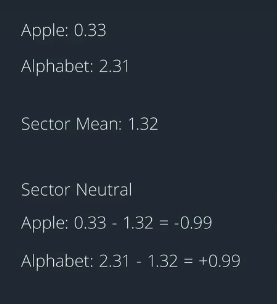# AI For Trading:Researching Alphas from Academic Papers (61)

We should not expect to get strong production-ready alphas "as is " from academic papers.

Publication erodes performance of the alpha model over time.

Why then do we look at academic papers at all ? For four reasons:

Reasons to study academic papers:

• 1、Idea generation
• 2、Baseline for comparison
• 3、New methods
• 4、New data

• General interest journals
• Investment journals
• Open source journals

Open source repositories:

• SSRN
• Archive

## Controlling for Risk within an alpha factor 1

Let's say we've read a paper, extracted some ideas, and have come up with an Alpha factor. Now, let's talk about some of the common early transformations we might do to that factor in order to move it closer towards our goal.

A vector of numbers that represents the weights we'd use to create a portfolio. These transformations help to enhance, improve, and prepare our Alpha factors so that they may be used during portfolio optimization to choose actual portfolio weights.

When we actually implement traits for our portfolio, we want that portfolio to be neutral to common risk factors. We should not wait however for the optimization step to think about common risk as we do not want to rely exclusively on optimization to make this happen.

Dollar neutral: Subtract the mean from each alpha value in the vector so that its values sum to zero.

## Controlling for Risk within an Alpha factor 2

So now that we've controlled for market risk,how can we control sector risk at this point. We often want our alpha factor to be sector neutral so that it's not exposed to overall movements within a sector and this means that we want our short weights in the sector to be equal in magnitude to our long waits in the sector.

We achieve this by calculating the average of all weights in the sector then subtracting the sector mean from each weight within that sector. Again, will make the simplifying assumption that each stock's Beta to its sector is equal to 1.

Let's look at a simple example with only two stocks in the tech sector; Apple an Alphabet. To make our alpha factor neutral to the tech sector, we could go along one of these stocks and short the other with the weight of the same magnitude. If we think about our raw Alpha factor, let's say that Apple's raw Alpha value is 0.33 and Alphabet's are raw alpha value is 2.31. The average is 1.32 and we subtract this mean from each stock's raw-value. So Apple's sector neutral Alpha value is now negative 0.99 and Alphabets is positive 0.99.

So by subtracting the industry average,we're able to convert this raw Alpha factor into an Alpha factor that is sector neutral. Again this is because the sum of the negative weights is equal to the magnitude of the sum of the positive weights in each sector. If the entire sector were to move up or down, the sector neutral portfolio would be unlikely affected by this movement.

A portfolio would likely consist of multiple sectors. So we'd repeat the sector neutralization for each sector.Note that we can neutralize the Alpha vector by market and also by sector. So in practice, we could first neutralized by the market and then take that re-scaled vector and neutralize it by sector.